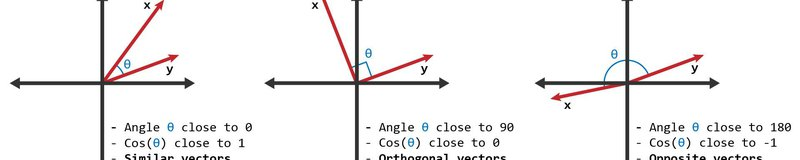# Cosine Similarity

## What is Cosine Similarity?

Cosine similarity is a metric used to measure the similarity of two vectors. Specifically, it measures the similarity in the direction or orientation of the vectors ignoring differences in their magnitude or scale. Both vectors need to be part of the same inner product space, meaning they must produce a scalar through inner product multiplication. The similarity of two vectors is measured by the cosine of the angle between them.

## How to calculate Cosine Similarity

We define cosine similarity mathematically as the dot product of the vectors divided by their magnitude. For example, if we have two vectors, A and B, the similarity between them is calculated as:

$$similarity(A,B) = cos(\theta) = \frac{A \cdot B}{\|A\|\|B\|}$$

where

• $\theta$ is the angle between the vectors,
• $A \cdot B$ is dot product between A and B and calculated as $A \cdot B = A^T B= \sum_{i =1} ^{n} A_iB_i = A_1B_1 + A_2B_2 + ...+ A_nB_n$,
• $\|A\|$ represents the L2 norm or magnitude of the vector which is calculated as $\|A\| = \sqrt{A_1^2 + A_1^2 ... A_1^n}$.

The similarity can take values between -1 and +1. Smaller angles between vectors produce larger cosine values, indicating greater cosine similarity. For example:

• When two vectors have the same orientation, the angle between them is 0, and the cosine similarity is 1.
• Perpendicular vectors have a 90-degree angle between them and a cosine similarity of 0.
• Opposite vectors have an angle of 180 degrees between them and a cosine similarity of -1.

Here's a graphic showing two vectors with similarities close to 1, close to 0, and close to -1.

## Applications

Cosine similarity is beneficial for applications that utilize sparse data, such as word documents, transactions in market data, and recommendation systems because cosine similarity ignores 0-0 matches. Counting 0-0 matches in sparse data would inflate similarity scores. Another commonly used metric that ignores 0-0 matches is Jaccard Similarity.

Cosine Similarity is widely used in Data Science and Machine Learning applications. Examples include measuring the similarity of:

• Documents in natural language processing
• Movies, books, videos, or users in recommendation systems
• Images in computer vision

## Numerical Example

Suppose that our goal is to calculate the cosine similarity of the two documents given below.

• Document 1 = 'the best data science course'
• Document 2 = 'data science is popular'

After creating a word table from the documents, the documents can be represented by the following vectors:

thebestdatasciencecourseispopular
D11111100
D20011011
• $D1 = [1,1,1,1,1,0,0]$
• $D2 = [0,0,1,1,0,1,1]$

Using these two vectors we can calculate cosine similarity. First, we calculate the dot product of the vectors:

$$D1 \cdot D2 = 1\times0+1\times0+1\times1+1\times1+1\times0+0\times1+0\times1=2$$

Second, we calculate the magnitude of the vectors: $$\|D1\| = \sqrt {1^2+1^2+1^2+1^2+1^2+0^2+0^2}=\sqrt5$$

$$\|D2\| = \sqrt {0^2+0^2+1^2+1^2+0^2+1^2+1^2}=\sqrt4$$

Finally, cosine similarity can be calculated by dividing the dot product by the magnitude $$similarity(D1, D2) =\frac{D1 \cdot D2}{\|D1\|\|D2\|} = \frac {2}{\sqrt5 \sqrt4} = \frac {2} {\sqrt{20}} = 0.44721$$

The angle between the vectors is calculated as:

$$cos(\theta) =0.44721$$ $$\theta = \arccos({0.44721}) = 63.435$$

## Python Example

We will use NumPy to perform the cosine similarity calculations.

Below, we defined a function that takes two vectors and returns cosine similarity. The Python comments detail the same steps as in the numeric example above.

import numpy as np

def cosine_similarity(x, y):

# Ensure length of x and y are the same
if len(x) != len(y) :
return None

# Compute the dot product between x and y
dot_product = np.dot(x, y)

# Compute the L2 norms (magnitudes) of x and y
magnitude_x = np.sqrt(np.sum(x**2))
magnitude_y = np.sqrt(np.sum(y**2))

# Compute the cosine similarity
cosine_similarity = dot_product / (magnitude_x * magnitude_y)

return cosine_similarity

Learn Data Science withAs an example, Cosine similarity will be employed to find the similarity between the following two documents:

corpus = [  'data science is one of the most important fields of science',
'this is one of the best data science courses',
'data scientists analyze data'   ]

Learn Data Science withUsing sklearn, we'll vectorize the documents:

from sklearn.feature_extraction.text import CountVectorizer

# Create a matrix to represent the corpus
X = CountVectorizer().fit_transform(corpus).toarray()

print(X)

Learn Data Science withOut:
[[0 0 0 1 1 1 1 1 2 1 2 0 1 0]
[0 1 1 1 0 0 1 0 1 1 1 0 1 1]
[1 0 0 2 0 0 0 0 0 0 0 1 0 0]]

Learn Data Science withWith the above vectors, we can now compute cosine similarity between the corpus documents:

cos_sim_1_2 = cosine_similarity(X[0, :], X[1, :])
cos_sim_1_3 = cosine_similarity(X[0, :], X[2, :])
cos_sim_2_3 = cosine_similarity(X[1, :], X[2, :])

print('Cosine Similarity between: ')
print('\tDocument 1 and Document 2: ', cos_sim_1_2)
print('\tDocument 1 and Document 3: ', cos_sim_1_3)
print('\tDocument 2 and Document 3: ', cos_sim_2_3)

Learn Data Science withOut:
Cosine Similarity between:
Document 1 and Document 2:  0.6885303726590962
Document 1 and Document 3:  0.21081851067789195
Document 2 and Document 3:  0.2721655269759087

Learn Data Science withAlternatively, Cosine similarity can be calculated using functions defined in popular Python libraries. Examples of such functions can be found in sklearn.metrics.pairwise.cosine_similarity (docs) and in the SciPy library's cosine distance fuction.

Here's an example of using sklearn's function:

from sklearn.metrics.pairwise import cosine_similarity

Learn Data Science withcos_sim_1_2 = cosine_similarity([X[0, :], X[1, :]])

print('Cosine Similarity between Document 1 and Document 2 is \n',cos_sim_1_2 )

Learn Data Science withOut:
Cosine Similarity between Document 1 and Document 2 is
[[1.         0.68853037]
[0.68853037 1.        ]]

Learn Data Science withThe results are same with the defined function. Notice that the input to sklearn's function is a matrix, and the output is also a matrix.